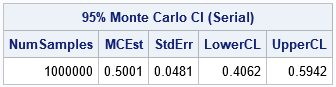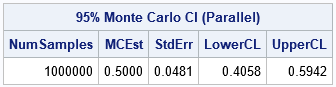A previous article introduces the MAPREDUCE function in the iml action. (The iml action was introduced in Viya 3.5.) The MAPREDUCE function implements the map-reduce paradigm, which is a two-step process for distributing a computation to multiple threads. The example in the previous article adds a set of numbers by distributing the computation among four threads. During the "map" step, each thread computes the partial sum of one-fourth of the numbers. During the "reduce" step, the four partial sums are added to obtain the total sum. The same map-reduce framework can be used to implement a wide range of parallel computations. This article uses the MAPREDUCE function in the iml action to implement a parallel version of a Monte Carlo simulation.

### Serial Monte Carlo simulation

Before running a simulation study in parallel, let's present a serial implementation in the SAS/IML language. In a Monte Carlo simulation, you simulate B random samples of size N from a probability distribution. For each sample, you compute a statistic. When B is large, the distribution of the statistics is a good approximation to the true sampling distribution for the statistic.

The example in this article uses simulation to approximate the sampling distribution of the sample mean. You can use the sampling distribution to estimate the standard error and to estimate a confidence interval for the mean. This example appears in the book Simulating Data with SAS(Wicklin 2013, p. 55–57) and in the paper "Ten Tips for Simulating Data with SAS" (Wicklin 2015, p. 6-9). The SAS/IML program runs a simulation to approximate the sampling distribution of the sample mean for a random sample of size N=36 that is drawn from the uniform U(0, 1) distribution. It simulates B=1E6 (one million) samples. The main function is the SimMeanUniform function, which does the following:

1. Uses the RANDSEED subroutine to set a random number seed.
2. Uses the RANDGEN subroutine to generate B samples of size N from the U(0, 1) distribution. In the N x B matrix, X, each column is a sample and there are B samples.
3. Uses the MEAN function to compute the mean of each sample (column) and returns the row vector of the sample means.

The remainder of the program estimates the population mean, the standard error of the sample mean, and a 95% confidence interval for the population mean.

```proc iml; start SimMeanUniform(N, seed, B); call randseed(seed); /* set random number stream */ x = j(N, B); /* allocate NxB matrix for samples */ call randgen(x, 'Uniform'); /* simulate from U(0,1) */ return mean(x); /* return row vector = mean of each column */ finish;   /* Simulate and return Monte Carlo distribution of mean */ stat = SimMeanUniform(36, /* N = sample size */ 123, /* seed = random number seed */ 1E6); /* B = total number of samples */   /* Monte Carlo estimates: mean, std err, and 95% CI of mean */ alpha = 0.05; stat = colvec(stat); numSamples = nrow(stat); MCEst = mean(stat); /* estimate of mean, */ SE = std(stat); /* standard deviation, */ call qntl(CI, stat, alpha/2 || 1-alpha/2); /* and 95% CI */ R = numSamples || MCEst || SE || CI`; /* combine for printing */ print R[format=8.4 L='95% Monte Carlo CI (Serial)' c={'NumSamples' 'MCEst' 'StdErr' 'LowerCL' 'UpperCL'}];```This program takes less than 0.5 seconds to run. It simulates one million samples and computes one million statistics (sample means). More complex simulations take longer and can benefit by distributing the computation to many threads, as shown in the next section.

### A distributed Monte Carlo simulation

Suppose that you have access to a cluster of four worker nodes, each of which runs eight threads. You can distribute the simulation across the 32 threads and ask each thread to perform 1/32 of the simulation. Specifically, each thread can simulate 31,250 random samples from U(0,1) and return the sample means. The sample means can then be concatenated into a long vector and returned. The rest of the program (the Monte Carlo estimates) does not need to change.

The goal is to get the SimMeanUniform function to run on all 32 available threads. One way to do that is to use the SimMeanUniform function as the mapping function that is passed to the MAPREDUCE function. To use SimMeanUniform as a mapping function, you need to make two small modifications:

• The function currently takes three arguments: N, seed, and B. But a mapping function that is called by the MAPREDUCE function is limited to a single argument. The solution is to pack the arguments into a list. For example, in the main program define L = [#'N'=36, #'seed' = 123, #'B' = 1E6] and pass that list to the MAPREDUCE function. In the definition of the SimMeanUniform module, define the signature as SimMeanUniform(L) and access the parameters as L\$'N', L\$'seed', and L\$'B'.
• The SimMeanUniform function currently simulates B random samples. But if this function runs on 32 threads, we want each thread to generate B/32 random samples. One solution would be to explicitly pass in B=1E6/32, but a better solution is to use the NPERTHREAD function in the iml action. The NPERTHREAD function uses the FLOOR-MOD trick to determine how many samples should be simulated by each thread. You specify the total number of simulations, and the NPERTHREAD function uses the thread ID to determine the number of simulations for each thread.

With these two modifications, you can implement the Monte Carlo simulation in parallel in the iml action. Because the mapping function returns a row vector, the reducer will be horizontal concatenation, which is available as the built-in "_HCONCAT" reducer. Thus, although each thread returns a row vector that has 31,250 elements, the MAPREDUCE function will return a row vector that has one million elements. The following program implements the parallel simulation in the iml action. If you are not familiar with using PROC CAS to call the iml action, see the getting started example for the iml action.

```/* Simulate B independent samples from a uniform(0,1) distribution. Mapper: Generate M samples, where M ~ B/numThreads. Return M statistics. Reducer: Concatenate the statistics. Main Program: Estimate standard error and CI for mean. */ proc cas; session sess4; /* use session with four workers */ loadactionset 'iml'; /* load the action set */ source SimMean; /* put program in SOURCE/ENDSOURCE block */   start SimMeanUniform(L); /* define the mapper */ call randseed(L\$'seed'); /* each thread uses different stream */ M = nPerThread(L\$'B'); /* number of samples for thread */ x = j(L\$'N', M); /* allocate NxM matrix for samples */ call randgen(x, 'Uniform'); /* simulate from U(0,1) */ return mean(x); /* row vector = mean of each column */ finish;   /* ----- Main Program ----- */ /* Put the arguments for the mapper into a list */ L = [#'N' = 36, /* sample size */ #'seed' = 123, /* random number seed */ #'B' = 1E6 ]; /* total number of samples */ /* Simulate on all threads; return Monte Carlo distribution */ stat = MapReduce(L, 'SimMeanUniform', '_HCONCAT'); /* reducer is "horiz concatenate" */   /* Monte Carlo estimates: mean, std err, and 95% CI of mean */ alpha = 0.05; stat = colvec(stat); numSamples = nrow(stat); MCEst = mean(stat); /* estimate of mean, */ SE = std(stat); /* standard deviation, */ call qntl(CI, stat, alpha/2 || 1-alpha/2); /* and 95% CI */ R = numSamples || MCEst || SE || CI`; /* combine for printing */ print R[format=8.4 L='95% Monte Carlo CI (Parallel)' c={'NumSamples' 'MCEst' 'StdErr' 'LowerCL' 'UpperCL'}];   endsource; /* END of the SOURCE block */ iml / code=SimMean nthreads=8; /* run the iml action in 8 threads per node */ run;```The output is very similar to the output from PROC IML. There are small differences because this program involves random numbers. The random numbers used by the serial program are different from the numbers used by the parallel program. By the way, each thread in the parallel program uses an independent set of random numbers. In a future article. I will discuss generating random numbers in parallel computations.

Notice that the structure of the parallel program is very similar to the serial program. The changes needed to "parallelize" the serial program are minor for this example. The benefit, however, is huge: The parallel program runs about 30 times faster than the serial program.

### Summary

This article shows an example of using the MAPREDUCE function in the iml action, which is available in SAS Viya 3.5. The example shows how to divide a simulation study among k threads that run concurrently and independently. Each thread runs a mapping function, which simulates and analyzes one-kth of the total work. (In this article, k=32.) The results are then concatenated to form the final answer, which is a sampling distribution.

For comparison, the Monte Carlo simulation is run twice: first as a serial program in PROC IML and again as a parallel program in the iml action. Converting the program to run in parallel requires some minor modifications but results in a major improvement in overall run time.

For more information and examples, see Wicklin and Banadaki (2020), "Write Custom Parallel Programs by Using the iml Action," which is the basis for these blog posts. Another source is the SAS IML Programming Guide, which includes documentation and examples for the iml action.

Share